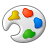# Getting a List of Values from a Field in a Feature class or Standalone table and comparing those values

173
2
06-30-2022 11:56 AMby
New Contributor II

Good afternoon,

I am trying to generate 2 separate lists for 2 different fields within a feature class/standalone and compare/sort them in such way that the order of the values from both fields are in ascending order. For example, using the selected values in the screenshot below, I want a separate list for BEGIN_DFO_MS and another for END_DFO_MS and then I want to be able to arrange/sort/compare the values that they line up in this manner:  123.506-128.149, 128.149-128.666, 128.666-129.598, 129.598-130.316.This is probably a lot of gibberish but I'm new to arcpro SDK and c# in general and would appreciate any help.

Thank you.

Tags (3)
1 Solution

Accepted SolutionsbyEsri Regular Contributor

there are a couple of ways I can think of to do the sort. One is to use the Geodatabase API and the SortDescription and TableSortDescription classes. Another way is to use LINQ OrderBy. LINQ also has a SortedList and various other collections optimized for sorting u could look at.

The advantage of using the GDB would be if your dataset is large. LINQ is probably quicker if your dataset is small. GDB sorting is further described here.

In this example I am using a feature class called "Crimes" containing some made up crime data where each incident has an X and Y coordinate stored in the attribute table which i will use to replicate your scenario.``````internal class SortFieldValues : Button {
protected async override void OnClick() {
var crimes = MapView.Active.Map.FindLayers("Crimes").First()
as FeatureLayer;
await QueuedTask.Run(() =>  {

var field_name = "X_Coordinate";
var field_name2 = "Y_Coordinate";
var asc_sort_list_x = new List<double>();
var asc_sort_list_y = new List<double>();

//Do the sort using GDB
using (var fc = crimes.GetFeatureClass()) {
using (var fc_def = fc.GetDefinition()) {
var x_field = fc_def.GetFields().First(f => f.Name == field_name);
var y_field = fc_def.GetFields().First(f => f.Name == field_name2);

//create a sort description for each field
var xSortDesc = new SortDescription(x_field);
var ySortDesc = new SortDescription(y_field);
//Sort order
xSortDesc.SortOrder = SortOrder.Ascending;
ySortDesc.SortOrder = SortOrder.Ascending;

//table sort
var TableSort_by_X = new TableSortDescription(
new List<SortDescription>() { xSortDesc });
var TableSort_by_Y = new TableSortDescription(
new List<SortDescription>() { ySortDesc });

//do the sorts
//x first
using (var rc = fc.Sort(TableSort_by_X)) {
while(rc.MoveNext()) {
if (rc.Current[x_field.Name] == null ||
rc.Current[x_field.Name] is DBNull)
continue;//TODO handle nulls as needed...
}
}
//now Y
using (var rc = fc.Sort(TableSort_by_Y)) {
while (rc.MoveNext()) {
if (rc.Current[y_field.Name] == null ||
rc.Current[y_field.Name] is DBNull)
continue;//TODO handle nulls...
}
}

//first 100
for(int i = 0; i < 100; i++) {
System.Diagnostics.Debug.WriteLine(
\$"X: {asc_sort_list_x[i]}  Y:{asc_sort_list_y[i]}");
}

asc_sort_list_x.Clear();
asc_sort_list_y.Clear();

//Do the sort using LINQ
using (var rc = fc.Search()) {
while (rc.MoveNext()) {
if (rc.Current[x_field.Name] == null ||
rc.Current[x_field.Name] is DBNull)
continue;//TODO handle nulls...

if (rc.Current[y_field.Name] == null ||
rc.Current[y_field.Name] is DBNull)
continue;//TODO handle nulls...

}
}

var result_w_linq_x = asc_sort_list_x.OrderBy(x => x).ToList();
var result_w_linq_y = asc_sort_list_y.OrderBy(y => y).ToList();

//first 100
for (int i = 0; i < 100; i++)
{
System.Diagnostics.Debug.WriteLine(
\$"X: {result_w_linq_x[i]}  Y:{result_w_linq_y[i]}");
}

}
}
});
}
}``````

2 RepliesbyEsri Regular Contributor

there are a couple of ways I can think of to do the sort. One is to use the Geodatabase API and the SortDescription and TableSortDescription classes. Another way is to use LINQ OrderBy. LINQ also has a SortedList and various other collections optimized for sorting u could look at.

The advantage of using the GDB would be if your dataset is large. LINQ is probably quicker if your dataset is small. GDB sorting is further described here.

In this example I am using a feature class called "Crimes" containing some made up crime data where each incident has an X and Y coordinate stored in the attribute table which i will use to replicate your scenario.``````internal class SortFieldValues : Button {
protected async override void OnClick() {
var crimes = MapView.Active.Map.FindLayers("Crimes").First()
as FeatureLayer;
await QueuedTask.Run(() =>  {

var field_name = "X_Coordinate";
var field_name2 = "Y_Coordinate";
var asc_sort_list_x = new List<double>();
var asc_sort_list_y = new List<double>();

//Do the sort using GDB
using (var fc = crimes.GetFeatureClass()) {
using (var fc_def = fc.GetDefinition()) {
var x_field = fc_def.GetFields().First(f => f.Name == field_name);
var y_field = fc_def.GetFields().First(f => f.Name == field_name2);

//create a sort description for each field
var xSortDesc = new SortDescription(x_field);
var ySortDesc = new SortDescription(y_field);
//Sort order
xSortDesc.SortOrder = SortOrder.Ascending;
ySortDesc.SortOrder = SortOrder.Ascending;

//table sort
var TableSort_by_X = new TableSortDescription(
new List<SortDescription>() { xSortDesc });
var TableSort_by_Y = new TableSortDescription(
new List<SortDescription>() { ySortDesc });

//do the sorts
//x first
using (var rc = fc.Sort(TableSort_by_X)) {
while(rc.MoveNext()) {
if (rc.Current[x_field.Name] == null ||
rc.Current[x_field.Name] is DBNull)
continue;//TODO handle nulls as needed...
}
}
//now Y
using (var rc = fc.Sort(TableSort_by_Y)) {
while (rc.MoveNext()) {
if (rc.Current[y_field.Name] == null ||
rc.Current[y_field.Name] is DBNull)
continue;//TODO handle nulls...
}
}

//first 100
for(int i = 0; i < 100; i++) {
System.Diagnostics.Debug.WriteLine(
\$"X: {asc_sort_list_x[i]}  Y:{asc_sort_list_y[i]}");
}

asc_sort_list_x.Clear();
asc_sort_list_y.Clear();

//Do the sort using LINQ
using (var rc = fc.Search()) {
while (rc.MoveNext()) {
if (rc.Current[x_field.Name] == null ||
rc.Current[x_field.Name] is DBNull)
continue;//TODO handle nulls...

if (rc.Current[y_field.Name] == null ||
rc.Current[y_field.Name] is DBNull)
continue;//TODO handle nulls...

}
}

var result_w_linq_x = asc_sort_list_x.OrderBy(x => x).ToList();
var result_w_linq_y = asc_sort_list_y.OrderBy(y => y).ToList();

//first 100
for (int i = 0; i < 100; i++)
{
System.Diagnostics.Debug.WriteLine(
\$"X: {result_w_linq_x[i]}  Y:{result_w_linq_y[i]}");
}

}
}
});
}
}``````by
New Contributor II

Thank you @CharlesCao . I will try out this solution and give feedback.